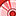# QlikView App Dev

Discussion Board for collaboration related to QlikView App Development.

Announcements
Our next Qlik Insider session will cover new key capabilities. Join us August 11th REGISTER TODAY
cancel
Showing results for
Did you mean:Contributor II

## Count Distinct for a time period

I am trying to get a sku count for a brand for the current year.  The problem is I get the total skus that are in the data set. I cannot figure out how to write the formula to only select sku count for the max year.   I tried the below formula to get only the sku count if sales were in the current year but it still results in total skus that were ever in the data set.  For example I should have 21 skus but it shows 43 because that is the maximum number of skus that have launched but only 21 have sales in the current year.

IF(sum({\$<IsInYTD={1},[Restated POS Year] = {\$(=Max([Restated POS Year]))}>} ZINVSLS >1), count(DISTINCT{\$<IsInYTD={1},[POS Year] = {\$(=Max([POS Year]))}>} Material))

3 RepliesSpecialist II

Try adding {1} to the Max function to look at all dates.

IF(sum({\$<IsInYTD={1},[Restated POS Year] = {\$(=Max({1}[Restated POS Year]))}>} ZINVSLS >1), count(DISTINCT{\$<IsInYTD={1},[POS Year] = {\$(=Max({1}[POS Year]))}>} Material))Contributor II
Author

I tried adding the adding {1} to the Max function to look at all dates and it returned the same value of 43.  If I use the sum of rows for total the sku count is correct.  If I use the expression total it is incorrect.  I need to display the data in a pivot and don't have the option to sum the rows in a pivot.Contributor II
Author

I found that count(distinct if ( (ZINVSLS)>1,Material)) returns the correct value if I select a certain year but when I try count(distinct if (sum({<Year={'2020'}>}(ZINVSLS)>1), Material)) I get an error.  I want my expression to calculate sku count for a specific year.Community Browser